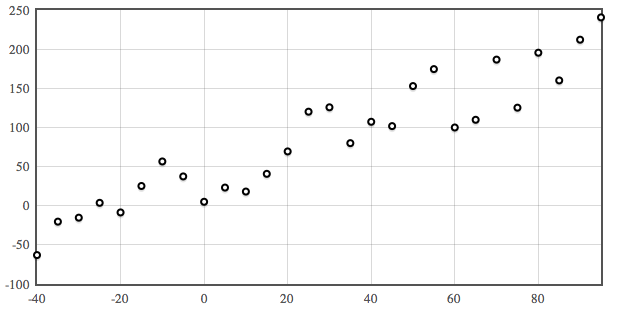Have a look at this picture. What do you notice? “It’s a straight line, Colin!” Very good. You could get a ruler out and draw a straight line through the points. Why would you bother doing such a thing? Well, the idea is that if you can model a data set - come up with a formula that describes it - then you can predict what would happen in hypothetical situations. This process is known as linear regression.

This particular straight line has the equation $F = 1.8 C + 32$ . If I wanted to predict the temperature in fahrenheit when I knew it was 28ºC outside, I could plug 28 in as C and get out an answer of 82.4ºF.… Which is all well and good when you have an immaculate straight line, but how about this one? Less of a straight line, certainly, but still a definite trend.

You could get your ruler out, certainly, and come out with a pretty decent line between the points. But there’s something deeply unsatisfying for a mathematician. Surely there’s a better way - a more accurate way - of finding the single line of best fit?

Well, of course. Otherwise I wouldn’t be writing this. Duh.

There are three ways (depending on the context) of working out the line of best fit. Quick GCSE reminder: a straight line needs a gradient (that you’ll remember being $m$) and a $y$-intercept (that you’ll remember being $c$). Statistics being statistics, it uses different letters: instead of $y = mx + c$ ((Which, of course, is the baby form of a straight line)), it uses $y = a + bx$. Your goal, when you do linear regression (which just means finding the line of best fit) is to work out $a$ and $b$.

The simplest way is to do it in Excel. I’ll do a screencast on how to do that another time, because you don’t have a computer in the exam. If you ask me, that’s stupid, but I’m not in charge of the world just now((Vote Colin for Supreme Leader if you think there should be computers in exams!))

### Linear regression on a calculator

If you have a Casio calculator, the kind with the round button in the middle at the top((The proper kind.)), you can get it to do the heavy lifting for you. This is the way I recommend doing it, because given the choice between adding up huge lists of numbers or letting a machine designed to add up huge lists of numbers, I’d generally leave it to the specialist.

Here’s what you do:

• Press mode and then ‘stat’, which is number 2 on my calculator. It’ll give you a table with $x$ and $y$ at the top of each column.
• Fill in your data, and read it back to make sure you haven’t missed or mistaken anything.
• Press ‘AC’ to get into normal calculator mode. It’ll say ‘STAT’ at the top, which is a Good Thing.
• Press shift then 1 to bring up the statistics menu. You want ‘regression’, which is 5 on my machine.
• It’ll give you a load of options - you want $a + bx$, which is number 2 for me.
• Oh look! There’s an $a$ and a $b$. I wonder what they are? Actually, I know what they are. They’re the $a$ and the $b$ from the equation. Press the number next to $a$ (1 for me) and then equals. It’ll give you the value of $a$.
• Go back to step 4 and do the same thing but press the number for $b$ in the last step. That’ll give you (ta-da!) $b$. The calculator has done the linear regression for you!

### Linear regression the hard way

That’s a lot easier than doing it the long way - which is to use the formulas in the formula book to work out $S_{xy}$ and $S_{xx}$; quite often in exam questions, you’re given handy numbers like $\sum x^2$ and $\sum xy$, just like you never are in real life.

Once you’ve worked those out ($S_{xx} = \sum(x^2) - \frac{\sum(x)^2}{n}$, and $S_{xy} = \sum(xy) - \frac{\sum(x)\sum(y)} {n}$), $b$ is just $\frac{S_{xy}}{S_{xx}}$. To find $a$, you need to know $\bar{x}$ (the mean of the $x$s) and $\bar{y}$ (surprisingly, the mean of the $y$s): $a = \bar{y} - b\bar{x}$ (so that the line goes through $(\bar{x}, \bar{y}$)((You could also write the equation as $y - \bar{y} = m (x - \bar x)$)).

And that’s it!